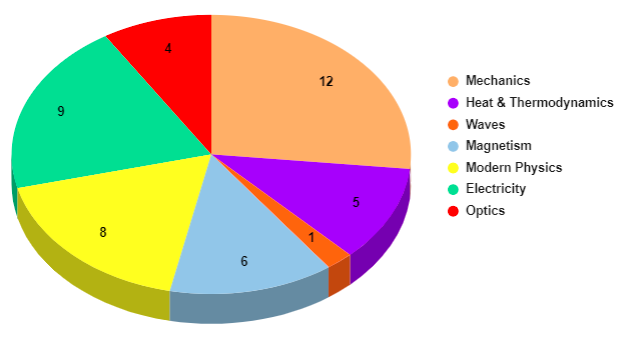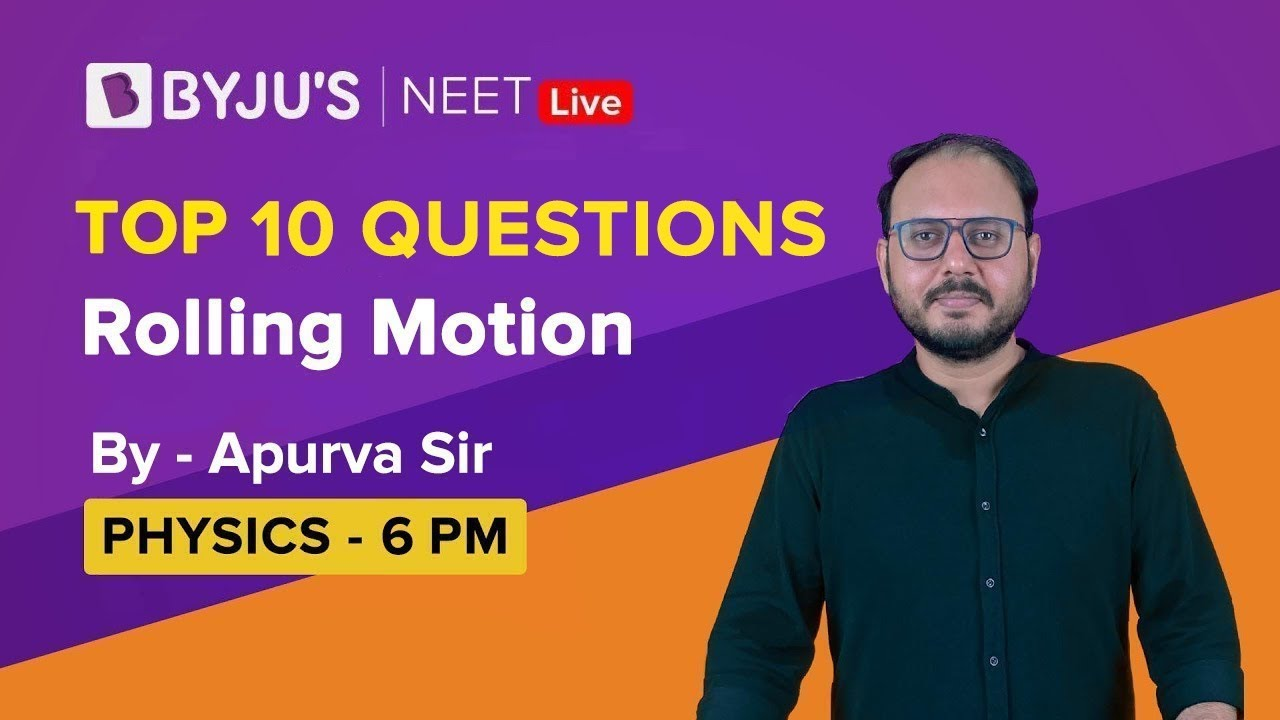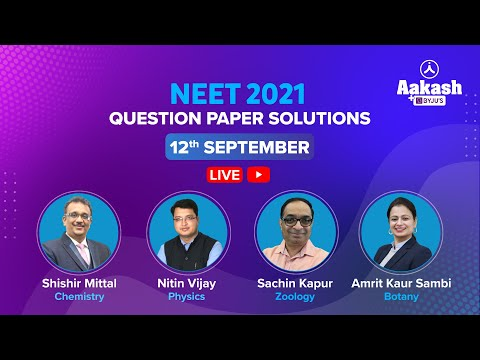# NEET Physics Syllabus for 2022

National Eligibility cum Entrance Test (NEET) will be carried out by the NTA. Knowledge of the syllabus will immensely help students to prepare for exams. The syllabus for NEET is provided after in-depth reviewing sessions by different states in addition to NCERT and COBSE (Council of Boards of School Education in India) as per the MCI (Medical Council of India). These measures are in view of maintaining uniformity while imparting medical education across India. NEET Physics Syllabus covers topics of both classes 11 and 12.

Also Check:

## Detailed NTA Prescribed NEET Physics Syllabus

### Class 11 NEET Physics Syllabus – Detailed Syllabus

Given Below is the list of all the topics included in class 11th NEET Physics syllabus chapterwise.

 Class 11 Physics Syllabus for NEET Physical world and measurement Kinematics Laws of motion Work, Energy and Power Motion of systems of particles and rigid body Gravitation Properties of bulk matter Thermodynamics Behaviour of Perfect gas and Kinetic theory Oscillations and Waves
 Unit 1 – Physical world and measurement Physics: scope and excitement; nature of physical laws; Physics, technology and society   Need for measurement – units of measurement; systems of units; SI units, fundamental and derived units. Length, mass and time measurements; accuracy and precision of measuring instruments; errors in measurement; significant figures   Dimensions of physical quantities, dimensional analysis and its applications Unit 2 – Kinematics Frame of reference, motion in a straight line; position-time graph, speed and velocity. Uniform and non-uniform motion, average speed and instantaneous velocity. Uniformly accelerated motion, velocity-time and position-time graphs for uniformly accelerated motion (graphical treatment) Elementary concepts of differentiation and integration for describing motion. Scalar and vector quantities: Position and displacement vectors, general vectors, general vectors and notation, equality of vectors, multiplication of vectors by a real number; addition and subtraction of vectors. Relative velocity Unit vectors. Resolution of a vector in a plane-rectangular components Scalar and vector products of vectors. Motion in a plane. Cases of uniform velocity and uniform acceleration – projectile motion. Uniform circular motion. Unit 3 – Laws of Motion Intuitive concept of force. Inertia, Newton’s first law of motion; momentum and Newton’s second law of motion; impulse; Newton’s third law of motion. Law of conservation of linear momentum and its applications Equilibrium of concurrent forces. Static and kinetic friction, laws of friction, rolling friction, lubrication Dynamics of uniform circular motion. Centripetal force, examples of circular motion (vehicle on level circular road, vehicle on banked road) Unit 4 – Work, Energy and Power Word done by a constant force and variable force; kinetic energy, work-energy theorem, power. Notion of potential energy, potential energy of a spring, conservative forces; conservation of mechanical energy (kinetic and potential energies); non-conservative forces; motion in a vertical circle, elastic and inelastic collisions in one and two dimensions. Unit 5 – Motion of systems of particles and rigid body Centre of mass of a two-particle system, momentum conservation and centre of mass motion. Centre of mass of a rigid; Centre of mass of uniform rod Momentum of a force – torque, angular momentum, conservation of angular momentum with some examples Equillibrium of rigid bodies, rigid body rotation and equation of rotational motion, comparision of linear and rotational motions; moment of inertia, radius of gyration. Values of M.I. for simple geometrical objects (no derivation). Statement of parallel and perpendicular axes theorems and their applications Unit 6 – Gravitation Kepler’s law of planetary motion. The universal law of gravitation. Acceleration due to gravity and its variation with altitude and depth Gravitational potential energy; gravitational potential. Escape velocity, orbital velocity of a satellite. Geostationary satellites Unit 7 – Properties of Bulk matter Elastic behaviour, stress-strain relationship. Hooke’s law, Young’s modulus, bulk modulus, shear, modulus of rigidity, poisson’s ratio; elastic energy Viscosity, Stoke’s law, terminal velocity, Reynold’s number, streamline and turbulent flow. Critical velocity, Bernoulli’s theorem and its applications. Surface energy and surface tension, angle of contact, excess of pressure, application of surface tension ideas to drops, bubbles and capillary rise Heat, temperature,thermal expansion; thermal expansion of solids, liquids and gases. Anomalous expansion. Specific heat of capacity:Cp, Cv-calorimetry; change of state-latent heat Heat transfer – conduction and thermal conductivity, convection and radiation. Qualitative ideas of Black body radiation. Wein’s displacement law, and green house effect. Newton’s law of cooling and Stefan’s law Unit 8 – Thermodynamics Thermal equilibrium and definition of temperature (zeroth law of thermodynamics). Heat, work and internal energy. First law of thermodynamics. Isothermal and adiabatic processes. Second law of the thermodynamics: Reversible and irreversible processes. Heat engines and refrigerators Unit 9 – Behaviour of perfect gas and kinetic theory Equation of state of a perfect gas, work done on compressing a gas Kinetic theory of gases: Assumptions, concept of pressure. Kinetic energy and temperature; degrees of freedom, law of equipartition of energy (Statement only) and application to specific heat capacities of gases; concept of mean free path Unit 10 – Oscillations and waves Periodic motion-period, frequency, displacement as a function of time. Periodic functions. Simple harmonic motion(SHM) and its equation; phase; oscillations of a spring-restoring force and force constant; energy in SHM – Kinetic and potential energies; simple pendulum – derivation of expression for its time period; free and forced and damped oscillations (qualitative ideas only), resonance Wave motion. Longitudinal and transverse waves, speed of wave motion. Displacement relation for a progressive wave. Principle of superposition of waves, reflection of waves, standing waves in strings and organ pipes, fundamental mode and harmonics. Beats. Doppler effect.

### Class 12 NEET Physics Syllabus – Detailed Syllabus

Given below is the list of all the topics included in class 12th NEET 2022 Physics syllabus chapterwise.

 NEET Physics Syllabus for Class 12 Electro statistics Current Electricity Magnetic Effects of Current and Magnetism Electromagnetic Induction and Alternating Currents Electromagnetic waves Optics Dual Nature of Matter and Radiation Atoms and Nuclei Electronic devices
 Unit 1 – Electrostatics Electric charges and their conservation. Coulomb’s law-force between two point charges, forces between multiple charges; superposition principle and continuous charge distribution   Electric field, electric field due to a point charge, electric field lines; electric dipole, electric field due to a dipole; torque on a dipole in a uniform electric field   Electric flux, statement of Gauss’s theorem and its applications to find field due to infinitely long straight wire, uniformly charged infinite plane sheet and uniformly charged thin spherical shell (field inside and outside)   Electric potential, potential difference, electric potential due to a point charge, a dipole and system of charges: equipotential surfaces, electrical potential energy of a system of two point charges and of electric dipoles in an electrostatic field   Conductors and insulators, free charges and bound charges inside a conductor. Dielectrics and electric polarization, capacitors and capacitance, combination of capacitors in series and in parallel, capacitance of a parallel plate capacitor with and without dielectric medium between the plates, energy stored in a capacitor, Van de Graaff generator Unit 2 – Current electricity Electric current, flow of electric charges in a metallic conductor, drift velocity and mobility, and their relation with electric current; Ohm’s law, electrical resistance, V-I characteristics (linear and non-linear), electrical energy and power, electrical resistivity and conductivity   Carbon resistors, colour code for carbon resistors; series and parallel combinations of resistors; temperature dependence of resistance.   Internal resistance of a cell, potential difference and emf of a cell, combination of cells in series and in parallel   Kirchoff’s laws and simple applications. Wheatstone bridge, metre bridge   Potentiometer-principle and applications to measure potential difference and for comparing emf of two cells; measurement of internal resistance of a cell Unit 3 – Magnetic effects of current and magnetism Concept of magnetic field, Oersted’s experiment. Biot-Savart law and its application to current carrying circular loop   Ampere’s law and its applications to infinitely long straight wire, straight and toroidal solenoids. Force on a moving charge in uniform magnetic and electric fields. Cyclotron   Force on a current-carrying conductor in a uniform magnetic field. Force between two parallel current-carrying conductors – definition of ampere. Torque experienced by a current loop in a magnetic field; moving coil galvanometer – its current sensitivity and conversion to ammeter and voltmeter   Current loop as a magnetic dipole and its magnetic dipole moment. Magnetic dipole moment of a revolving electron. Magnetic Field Intensity due to a magnetic dipole(bar magnet) along its axis and perpendicular to its axis. Torque on a magnetic dipole (bar magnet) in a uniform magnetic field; bar magnet as an equivalent soilenoid, magnetic field lines; Earth’s magnetic field; bar magnet as an equivalent solenoid, magnetic field lines; Earth’s magnetic  field and magnetic elements. Para -, dia-and ferro-magnetic substances, with examples.   Electromagnetic and factors affecting their strengths. Permanent magnets Unit 4 – Electromagnetic induction and alternating current Electromagnetic induction; Faraday’s law, induced emf and current; Lenz’s law, eddy currents. Self and mutual inductance   Alternating currents, peak and rms value of alternating current/voltage; reactance and impedance; LC oscillations (qualitative treatment only), LCR series circuit, resonance; power in AC circuits, wattles current   AC generator and transformer Unit 5 – Electromagnetic waves Need for displacement current   Electromagnetic waves and their characteristics (qualitative ideas only). Transverse nature of electromagnetic waves   Electromagnetic spectrum (radio waves, micro waves, infrared, visible, ultraviolet, x-rays, gamma rays) including elementary facts about their uses. Unit 6 – Optics Reflection of light, spherical mirrors, mirror formula. Refraction of light, total internal reflection and its applications optical fibers, refraction at spherical surfaces, lenses, thin lens formula, lens-maker’s formula. Magnification, power of a lens, combination of thin lenses in contact combination of a lens and a mirror. Refraction and dispersion of light through a prism   Scattering of light – blue color of the sky and reddish appearance of the sun at sunrise and sunset   Optical instruments: Human eye, image formation and accommodation, correction of eye defects (myopia and hyper myopia) using lenses   Microscopes and astronomical telescopes (reflecting and refracting) and their magnifying powers.   Wave optics: Wavefront and Huygen’s principle, reflection and refraction of plane wave at a plane surface using wavefronts   Proof of laws of reflection and refraction using Huygen’s principle   Interference, Young’s double hole experiment and expression for fringe width, coherent sources and sustained interference of light   Diffraction due to a single slit, width of central maximum   Resolving power of microscopes and astronomical telescopes. Polarisation, plane polarized light; Brewster’s law, uses of plane polarized light and polaroids Unit 7 – Dual nature of matter and radiation Photoelectric effect, Hertz and Lenard’s observations; Einstein’s photoelectric equation – particle nature of light Matter waves – wave nature of particles, deBrogile relation. Davisson-Germer experiment (experimental details should be omitted; only conclusion should be explained) Unit 8 – Atoms and nuclei Alpha – particle scattering experiments; Rutherford’s model fo atom; Bohr model, energy levels, hydrogen spectrum. Composition and size of nucleus, atomic masses, isotopes, isobars; isotones   Radioactivity – alpha, beta and gamma particles/rays and their properties decay law. Mass-energy relation, mass defect; binding energy per nucleon and its variation with mass number, nuclear fission and fusion Unit 9 – Electronic devices Energy bands in soilds (qualitative ideas only), conductors, insulators and semiconductors; semiconductor diode- I-V characteristics in forward and reverse bias, diode as a rectifier; I-V characteristics of LED, photodiode, solar cell, and Zener diode; Zener diode as a voltage regulator. Junction transistor, transistor action, characteristics of a transistor; transistor as an amplifier (Common emitter configuration) and oscillator. Logic gates (OR, AND, NOT, NAND and NOR)

### NEET Physics Syllabus Analysis:

Aspirants getting ready for NEET must start preparing with the most important topics in the syllabus. It is suggested to examine the weightage of all chapters in the NEET Physics Syllabus.

#### NEET 2021 Question Paper Analysis – Physics

 Chapters Questions Breakup Modern Physics & Electronics 8 Magnetism 7 SHM & Wave 3 Optics 4 Electrostatics and Electricity 11 Heat & Thermodynamics 4 Mechanics 13

The table below indicates the chapters and the corresponding marks they carry. The last column depicts the average number of questions, cumulatively taken, on the basis of previous years’ trends from the year 2006 onwards up till NEET 2019. Take a look at the table below:

 Name of Unit Chapter’s name Average no. of questions Physical World and Measurement Physical World and Units and Measurement 1 Kinematics Motion in Straight Line 2 Motion in a Plane 1-2 Laws of Motion Laws of Motion 2-3 Work, Energy and Power Work, Energy and Power 2 System of Particles and Rotational Motion System of Particles and Rotational Motion 2-3 Gravitation Gravitation 2 Properties of Bulk Matter Mechanical Properties of Solids 1 Mechanical Properties of Fluids 1 Thermal Properties of Matter 1-2 Oscillations and Waves Oscillations 1-2 Waves 1-2 Thermodynamics Thermodynamics 1-2 Behaviour of Perfect Gas and Kinetic Theory Kinetic Theory 1 Electrostatics Electric Charges and Fields 1-2 Electrostatic Potential and Capacitance 1-2 Current Electricity Current Electricity 4 Magnetic Effects of Current and Magnetism Moving Charges and Magnetism 2 Magnetism and Matter 1 Electromagnetic Induction and Alternating Currents Electromagnetic Induction 1 Alternating Currents 1 Electromagnetic Waves Electromagnetic Waves 1 Optics Ray Optics and Optical Instruments 2 Wave Optics 1 Dual Nature of Radiation and Matter Dual Nature of Radiation and Matter 2-3 Atoms and Nuclei Atoms 1-2 Nuclei 2 Electronic Devices Semiconductor Electronics 3 Communication Systems Communication Systems 0

The following table indicates the NEET 2020 Weightage of important chapters. Take a look:

NEET 2020 Weightage – Number Of Questions Asked

 Class Chapter name Number Of Questions Class 11 Mechanics 12 Heat & Thermodynamics 5 Waves 1 Class 12 Magnetism 6 Modern Physics 8 Electricity 9 Optics 4If one analyzes the NEET previous years Question papers, one can deduce the most important topics in the NEET Physics syllabus which are Mechanics, Kinematics, Heat, Electrostatics, Electronic Devices, Thermodynamics, The Magnetic effect of Current, Laws of Motion, and Oscillations & Waves.

More here: NEET Quiz Videos

## Important Chapters in NEET Physics

• Modern Physics – EM Waves, Dual nature of waves and particles, Atoms, Nuclei, Semiconductors
• Thermal Physics – Thermal properties of matter, Thermodynamics, Kinetic theory of gases
• Current electricity
• Optics – Ray optics, wave optics
• Electrostatics – Electric charges and fields, Electrostatic potential, Capacitor
• Kinematics – Vectors, Motion in one dimension and motion in two dimension

### NEET Physics Important Chapters – Classwise

Class 11 NEET Physics Important Chapters

• Units and measurements
• Motion in one dimension
• Laws of motion
• Work, Power and energy
• Gravitation
• Heat and Thermodynamics
• SHM

Class 12 NEET Physics Important Chapters

• Capacitor
• Magnetism
• Electromagnetic Wave
• Electromagnetic Induction
• Current electricity
• Alternating current
• Wave optics
• Modern Physics
• Semiconductors

### Tips to prepare with NEET Physics Syllabus 2022:

1. Syllabus and Exam Pattern Comprehension:

Analysing topics in NEET 2022 Physics Syllabus must be the first step while starting to prepare. Comprehend the exam pattern and hence the marking scheme.

2. Refer to Best Books:

NCERT physics books are one of the best books to prepare for exams. Several times questions are picked directly from the concepts taught in the NCERT textbooks. For strengthening your numerical portion, you may refer to other books as well.

A. H.C. Verma Concepts of Physics  (Volume 1 and 2)

B. Concepts of Competition Physics by Aggarwals (for CBSE PMT)

3. Practice Previous Year’s Question Papers:

One of the best ways to prepare for the NEET Physics Syllabus is to practice previous years’ NEET question papers. Solving these papers will help you to understand the type of questions asked in the examination. Also, it will enhance your speed, accuracy, time management skills, and familiarize you with the topics important from the examination point of view.

4. Make Proper Notes:

The students are advised to prepare study notes of all the necessary formulas, theorems, and concepts. These revision notes will help students to strengthen their knowledge and boost their last-minute preparations.

5. Appear for Online Mock Test Series:

Online Mock Test Series will help to improve your exam-taking skills. Taking Online Mock Tests will assist you to evaluate your preparation level. Moreover, it will also help you to identify your weak concepts.

Stay tuned with BYJU’S to know more about NEET preparation tips, NEET Physics syllabus weightage, NEET Physics syllabus pdf, study material, MCQs, and more.

Recommended Question Papers for NEET Exam## Frequently Asked Questions on NEET Physics Syllabus

### Are NEET Physics syllabus 2019, NEET Physics syllabus 2020 and NEET Physics syllabus 2021 all the same?

So far, the syllabus for NEET Physics has been the same. Since there has been a delay in the announcement of dates for NEET for different events and even the question of NEET 2021 getting postponed, it is likely that there might be a slight change in the syllabus. However, official notification on the same has not been out yet. So, the syllabus as of now remains the same.

### Is NEET Physics syllabus tough?

NEET tests medical aspirants in Physics, Chemistry and Biology subjects for knowledge of NCERT syllabus for NEET majorly. Given the tough competition and the ever-increasing interest amongst students opting for medicine as a career, the question paper over the years has been deemed to be challenging. Having said that, all sections of NEET are equally challenging in their own ways, Physics section requires more attention and practice from students’ end as it requires them to apply learnt concepts and solve questions. So, answering the question, NEET Physics could be challenging if one does not have a strong grip on basic concepts.